Courses

# Test: Circles- 1

## 15 Questions MCQ Test Quantitative Aptitude for GMAT | Test: Circles- 1

Description
This mock test of Test: Circles- 1 for GMAT helps you for every GMAT entrance exam. This contains 15 Multiple Choice Questions for GMAT Test: Circles- 1 (mcq) to study with solutions a complete question bank. The solved questions answers in this Test: Circles- 1 quiz give you a good mix of easy questions and tough questions. GMAT students definitely take this Test: Circles- 1 exercise for a better result in the exam. You can find other Test: Circles- 1 extra questions, long questions & short questions for GMAT on EduRev as well by searching above.
QUESTION: 1

Solution:
QUESTION: 2

Solution:
QUESTION: 3

###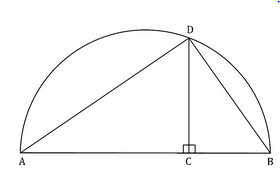In the given figure, the arc ADB is a semi-circle and the length of AD is 16cm and AB is 20cm. Find the length of CD?

Solution:
QUESTION: 4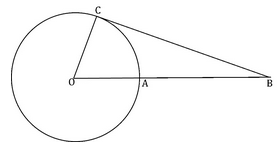In the given figure, O is the center of the circle and BC is the tangent. If AB = 10cm. Find the length of the tangent BC.

1. The product of the length of OB and AB is 170.
2. The circumference of the circle is 14π.
Solution:
QUESTION: 5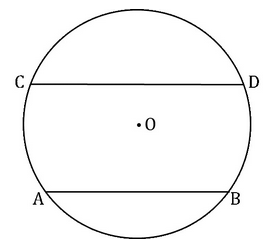AB and CD are two parallel chords in a circle with centre O and radius 5cm. If the length of AB = 6cm, CD = 8cm. Find the perpendicular distance between the two chords.

Solution:
QUESTION: 6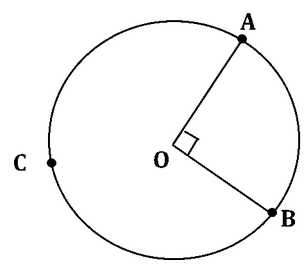If point O is the center of the circle as shown in the figure, what is the area of the circle (in sq. cm.) ?

(1) Length of the major arc ACB is 9π cm.

(2) Area of ΔOAB = 18 sq. cm.

Solution:

Approach

Steps 1 & 2: Understand Question and Draw Inferences

The figure shows the sector AOB that subtends an angle of 90o at the center of the circle.

Therefore ACB is the major arc that subtends an angle of 270o at the center.

We need to find the area of the circle. For this we need to find the radius of the circle.

Step 3: Analyze Statement 1

Given that length of major arc ACB is 9π cm.

Note that the ratio of arc lengths is equal to the ratio of angle subtended by the arcs at the center of the circle.

Let the circumference of the circle be C. (Note that the circle is essentially an arc subtending 360o at the center.)

Therefore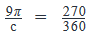⇒c=12π

Let us assume the radius as r cm.

Therefore, 2πr = 12π

⇒  r = 6 cm.

We can calculate the area by using the formula A = πr2

We get a unique answer.

So statement 1 is sufficient to arrive at a unique answer.

Step 4: Analyze Statement 2

ΔOAB is right angled with OA and OB as the perpendicular sides. (Note that both OA and OB are radii of the circle).

We know that in a right angled triangle, the area of the triangle is equal to ½*(product of the perpendicular sides).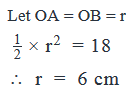We can calculate the area by using the formula A = πr2

We get a unique answer.

So statement 2 is sufficient to arrive at a unique answer.

Step 5: Analyze Both Statements Together (if needed)

We arrived at a unique answer in both Step 3 and Step 4 above. So this step is not required.

Correct Answer: D

QUESTION: 7

A circular track needs to be fenced along the inner and outer boundaries of the track to prevent stalkers from interrupting any events. What is the width of the track?

(1) The fencing used for the outer boundary can actually be used to fence the inner boundary twice.
(2) The area of the circle formed by the inner boundary is 10 sq. meter.

Solution:

Steps 1 & 2: Understand Question and Draw Inferences

Assume that the inner radius, outer radius and width of the circular track are r, R and w.

Therefore R = r + w

We need to find w.

w = R - r

So we need the values of r and R to find W.

Step 3: Analyze Statement 1

This statement says that the fencing used for outer boundary (perimeter of the outer boundary) is twice the size of the fencing for inner boundary (perimeter of inner boundary).

Perimeter of outer boundary = 2πR

Perimeter of inner boundary = 2πr

Therefore,

2πR = 2*2πr

R = 2r.

However, this information is not enough to find w.

Therefore statement 1 is not sufficient to arrive at a unique answer.

Step 4: Analyze Statement 2

The area of the inner circle is 10 sq. meters.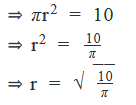But we still don’t know R and so we cannot calculate the value of w.

Step 5: Analyze Both Statements Together (if needed)

Combining the information we got from statements (1) and (2);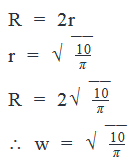Therefore statement (1) and statement (2) together are sufficient to arrive at a unique answer.

Correct Answer: C

QUESTION: 8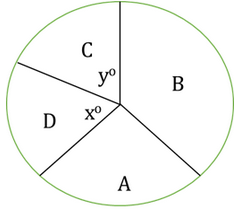A company X has offices in four locations – A, B, C and D. The figure above represents a circle graph of X’s profits from each of the four locations in April 2010. One-thirds of companies profits in were registered from B while one-fourths of its profits were registered from A. Were the profits from the location C of the company more than the profits from location D of the company?

(1) x > y

(2) The sum of the profits from A and D combined were larger than the sum of the profits from B and C combined.

Solution:

Steps 1 & 2: Understand Question and Draw Inferences

Since one-thirds of the total profits > one-fourths of the total profits,

the area of region B > area of region A

The question is:

Is the area of region C > area of region D?

Step 3: Analyze Statement 1

Since O is the centre of the circle and x > y,

area of region D > area of region C. (For two sectors in a circle, the sector with the greater angle at the centre has the greater area)

Therefore, the profits from company C were NOT greater than the profits from company
D.

SUFFICIENT.

Step 4: Analyze Statement 2

According to this statement

area of region A + area of region D > area of region C + area of region B

Since area of region A is smaller than area of region B, for this inequality to hold true, the area of region D must be larger than area of region C.

Therefore, the profits from company C were NOT greater than the profits from company D.

SUFFICIENT.

Step 5: Analyze Both Statements Together (if needed)

Since we have obtained an answer, there is no need to combine the statements.

QUESTION: 9

The floor of a rectangular room of 72 square meters area needs to be covered by a circular carpet that occupies exactly half the area of the floor. What must be the circumference of the carpet in meters?

Solution:

Given that the area of the rectangular floor = 72 square meters

And that the carpet occupies exactly half the area.

Area of carpet = 36 square meters.

Let the radius of carpet = R meters

Therefore πR2 = 36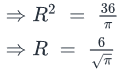Therefore the circumference  =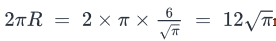meters

QUESTION: 10

Little Johnny wants to eat a candy (circular in shape) using chopsticks! For this he makes sure that one end of either chopstick is touching the other (held in his hand) and the other end is used to hold the candy, as shown in the figure below. If his mom wants him to maintain a distance of at least 10 cm between his hands and his mouth while eating, what is the minimum length (in cm) of chopsticks required by him to eat the candy using chopsticks? (Assume that both the chopsticks are of equal length and that the radius of the candy is 6 cm.)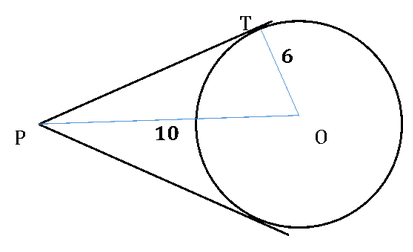Solution: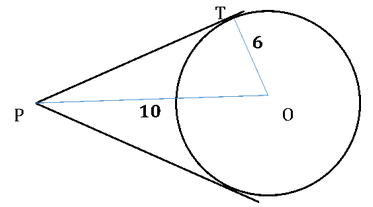From the given information we can see that the chopsticks act as tangents to the circular candy (As shown in the above figure.)

ΔOTP is right angled with OT and PT as the perpendicular sides. Therefore applying Pythagoras theorem on ΔOTP.

102 = 62 + PT2

⇒PT2 = 100 – 36 = 64

⇒PT = 8

Therefore the chopsticks must be of at least 8 cm length.

Correct Answer: A

QUESTION: 11

A man distributed his wealth among his wife and three sons such that his second son got twice the amount the eldest son received, his youngest son received twice the amount the second son got and his wife got twice the amount the youngest son received. If the man were to represent his wealth in a circle diagram, how many degrees of the circle must be allocated to represent the portion that the second son receives?

Solution:

Let the amounts of wealth received by the eldest son, second son, youngest son and the wife be E, S, Y and W respectively.

Given that

S = 2E

Y = 2S

W = 2Y

So if we assume that Eldest son got “x”, we have

E = x; S = 2x; Y = 4x; W = 8x;

and the total wealth = x + 2x + 4x + 8x = 15x.

So the fraction of wealth received by the second son is 2/15

So if we represent it on a circle (over 360o), the fraction would be converted to 215×360o=48o

Correct Answer: C

QUESTION: 12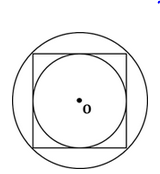A square is inscribed in a big circle and a small circle is inscribed in the square as shown in the figure above. (All of them are concentric and their center is the point O). If the radius of the big circle is √2 cm, what is the semi perimeter of the small circle?

Solution:

Let us assume that

the radius of big circle = R

side of the square = S

radius of the small circle = r

Observe that the diameter of the big circle = diagonal of the square.

• 2R = √2 * S
• S = √2 * R

Also the diameter of the small circle = side of the square

• 2r = S
• 2r = √2 * R

​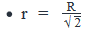Semi perimeter of the small circle = πr = π(R/√2)

Putting R = √2 in the above equation, we get:

Semi perimeter of the small circle = π

Correct Answer: D

QUESTION: 13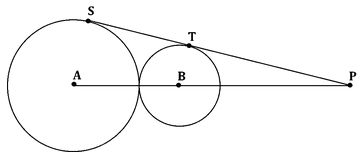A bigger circle (with center A) and a smaller circle (with center B) are touching each other externally. PT and PS are the tangents drawn to these circles from an external point (as shown in the figure). What is the length of ST?

(1) The radii of the bigger and the smaller circles are 9 cm and 4 cm respectively
(2) PB = 52/5 cm

Solution:

Steps 1 & 2: Understand Question and Draw Inferences

Given that PT is a tangent to the small circle and PS is a tangent to the big circle.

ΔPTB and ΔPSA are right angled at T and S respectively.

We need to find the length of ST.

Step 3: Analyze Statement 1

(1) The radii of the bigger and the smaller circles are 9 cm and 4 cm respectively.

If we drop a perpendicular BD on side AS, we get: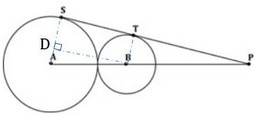BDST is a rectangle (since all angles of this quadrilateral are right angles).

Therefore, since opposite sides of a rectangle are equal, SD = BT = 4 cm

This means, AD = 9 – 4 = 5cm

In right triangle ADB, by applying Pythagoras Theorem, we get:

BD2 + AD2 = AB2

That is, BD2 = (9+4)2 – (5)2

BD2 = (13+5)(13-5)

BD2 = 18*8 = 24*32

Therefore, BD = 22*3 = 12

Since opposite sides of a rectangle are equal, ST = BD = 12 cm

Since we have been able to determine a unique length of ST, Statement (1) is sufficient.

Step 4: Analyze Statement 2

(2) PB =  52/5 cm

In right triangle BTP, we know the length of only one side: BP.

In order to find the lengths of the other sides of this triangle, we need one more piece of information – either one of the two unknown angles, or one of the two unknown sides.

Since we don’t have this information, we will not be able to find the lengths of the unknown sides of triangle BTP.

Due to a similar reasoning, we will not be able to find the length of the sides of triangle ASP.

So, we will not have enough information to find the length of ST.

Therefore statement 2 is not sufficient to arrive at a unique answer.

Step 5: Analyze Both Statements Together (if needed)

We have arrived at a unique answer in step 3 above. Hence this step is not required.

Correct Answer: A

QUESTION: 14

What is the area of the 144o sector of the circle?

(1) The square with one of the sides as the chord that subtends an angle of 90o on the circle measures 64 sq. cm. in area.
(2) When an isosceles triangle is constructed by taking the longest chord of the circle and the other vertex on the circumference of the circle, the length of each of the equal sides of the triangle is 4√2 cm.

Solution:

Steps 1 & 2: Understand Question and Draw Inferences

We need to find the area of a sector subtending an angle of 144o at the center of the circle.

For this we need either the radius of the circle or the area of a known portion of the circle.

Step 3: Analyze Statement 1

The chord that subtends an angle of 90o on the circle is the diameter itself.

(Please note that the chord here subtends an angle of 90o on the circle, and not on the center of the circle)

Area of the square with the side as the diameter is 64 sq. cm.

Therefore the length of the diameter = √(64) = 8 cm.

• Radius of the circle = 4 cm.

We can find the area of the 144o sector by using the formula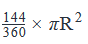Therefore statement 1 is sufficient to arrive at a unique answer.

Step 4: Analyze Statement 2

The longest chord of the circle is the diameter itself.

We know that the diameter subtends an angle of 90o on the circle.

Therefore the isosceles triangle constructed would be an isosceles right angled triangle with the diameter as the hypotenuse.

Given that the length of the equal sides is 4√2 cm.

Let the diameter = D

Therefore, D2 = (4√2)2 + (4√2)2 (Applying Pythagoras)

⇒  D2 = 64

⇒  D = 8 cm.

⇒  Radius = 4 cm.

We can find the area of the 144o sector by using the formulaTherefore statement 2 is sufficient to arrive at a unique answer.

Step 5: Analyze Both Statements Together (if needed)

We arrived at a unique answer in both Step 3 and Step 4 above. So this step is not required.

Correct Answer: D

QUESTION: 15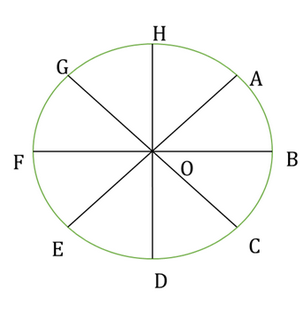The circle shown in the figure above is divided into eight parts, each of whose area is 8π. What is the total length of the boundary AOBCODEOFGOHA? (Note: If two points are connected by an arc, consider only the arc length. For example, DE represents arc DE while OB represents the line segment OB)

Solution:

Step 1: Question statement and Inferences

Since each part has equal area measuring 8π square units,

1/8 * π * r2 = 8π

r = 8

The circle is divided into eight equal arcs, i.e.

AB = BC = CD = DE = EF = FG = GH = HA

length of each arc = 1/8 * 2 * π * r

Substituting r = 8, we have

length of each arc = 2π

Step 2:Finding required values

length of boundary AOBCODEOFGOHA = radius AO + radius OB + arc BC + radius CO + radius OD + arc DE + radius EO + radius OF +  arc FG + radius GO + radius OH + arc HA

length of boundary AOBCODEOFGOHA = 8 * radius + 4 * length of arc

Step 3: Calculating the final answer

Substituting the values of radius and length of arc obtained above,

length of boundary AOBCODEOFGOHA = 8 * 8 + 4 * 2 π  = 64 + 8 π

Correct Answer: E